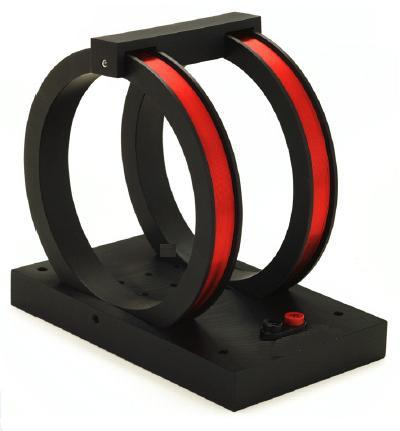#### products categories

• Tel : 18030236818
• Fax : +86-592 5237901
• Email : dexing@china-dexing.com

# Introduction To The Principle And Application Of Helmholtz Coils

According to the shape of the coil, the Helmholtz coil can be divided into a round Helmholtz coil and a square Helmholtz coil. According to the direction of the magnetic field, the Helmholtz coil can be divided into a one-dimensional Helmholtz coil, a two-dimensional helmholtz pair, and a three-dimensional Helmholtz coil. According to the properties of coil current, Helmholtz coils can be divided into DC Helmholtz coils and AC Helmholtz coils.

Helmholtz coil, uniform area volume, open space, easy to operate, with it can produce a very weak magnetic field up to hundreds of Gauss magnetic field; Can realize one-dimensional, two-dimensional, three-dimensional magnetic field combination, can provide AC magnetic field or DC magnetic field proportional to the coil current; Its internal space can be matched with a suitable non-magnetic support platform, easy for users to place the instrument or experimental equipment under test, very suitable for the research institute, universities and enterprises to do material magnetic or testing experiments, applied to materials, electronics, biology, medical, aerospace, chemistry, applied physics, and other disciplines.## What is special about Helmholtz coil?

A Helmholtz coil is a device that produces a uniform magnetic field over a small area. Due too the open nature of the Helmholtz coil, other instruments can be easily inserted or removed, and direct visual observation can be done, so it is often used in physical experiments devices.

Features: Open space, easy to use; There is a good linear relationship between the magnetic field and the supply current. There is a wide uniform area in the magnetic field space; Suitable for manufacturing 1D, 2D and 3D space combined magnetic field between two coils.

## What are Helmholtz coils used for?

A Helmholtz coil is a coil combination consisting of one or more pairs of coils with the same number of coil turns, the same coil winding method and the coil radius equal to the coil spacing. In industry, national defense, scientific research need to measure the magnetic field, there are many methods to measure the magnetic field, such as impact galvanometer method, Hall effect method, nuclear magnetic resonance method, balance method, helmholtz magnet induction method and so on.

A Helmholtz coil is a device that creates a uniform magnetic field in a small area. Since the Heimholtz coil is open, other instruments can be easily placed or removed, and visual observations can be made directly, so it is a commonly used device in physics experiments.

application of helmholtz coil:

Generate standard magnetic field between helmholtz coils (magnetic field generator)

Offset and compensation of the earth's magnetic field;

Geomagnetic environment simulation;

Determination of magnetic shielding effect;

Electromagnetic interference simulation experiment;

Calibration of Hall probe and various magnetometers;

Mobile phone magnetic compass algorithm calibration;

Study of biological magnetic field and magnetic properties of matter.

## What is the principle of Helmholtz coil?

The theory of the magnetic field produced by the Helmholtz coil is based on the BIOt-Savart law, which is applicable to the calculation of the magnetic field produced by a steady current. This current is the continuous flow of electric charge through a wire. The flow rate does not change with time. The charge does not accumulate or disappear anywhere.

A pair of coils whose axial distance is equal to the coil radius constitutes magnetic field of two helmholtz coils When the coils are connected in series and pass through a stable direct current, a relatively uniform axial magnetic field can be generated in the central area of the coil. Conversely, the parameters of a permanent magnet placed inside a Helmholtz coil can also be measured.

## Why do we use 2 coils in Helmholtz?

Let's first look at the distribution of the magnetic field of an electrified circle. According to the Biot-Savart law, it can be obtained by integration operation; The magnetic field is B= U *R2*I/2[R2+X2][3/2] on the axis over the center of the circle and perpendicular to the coil plane, where I is the current, R is the radius of the circle, and u is a constant. Helmholtz coils are two coaxial circular coils parallel to each other and connected. The magnetic field distribution of Helmholtz coils is the superposition of the magnetic fields of two energized circles. Depending on the radius and the distance between the two circles, the superposition results in different results. Outside the two coils, it gradually decreases, but between the magnetic field of two coils, it can be the weakest in the middle, it can be the strongest in the middle, and it can also be a uniform magnetic field.

## How many turns in a Helmholtz coil?

Typical cases of Helmholtz coil system, detailed technical parameters of three-dimensional helmholtz pair of coils:

1. The coil is made of electric wood, aluminum and PVC as the skeleton material of the coil

2. X coil: the winding diameter is 1.0, the physical diameter is about 140mm, the coil turns are 616, the coil resistance is about 12.35ohm, and the coil resistance is 150GS

3, Y coil: winding wire diameter 1.0, physical diameter 235mm, coil turns 1024, coil resistance about 34.8 ohms, 2A corresponding to 150Gs

4. Z coil: winding wire diameter 1.2, physical diameter about 380mm, coil turns 1672, coil resistance about 62 ohms, 2A corresponding to 150Gs